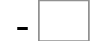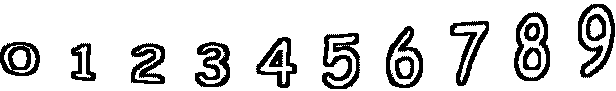15= 0   17= 650= 15   64= 40   70= 46   11= 1 58= 27   84= 34   90= 50   62= 24